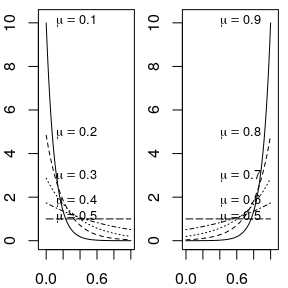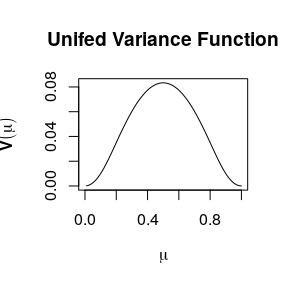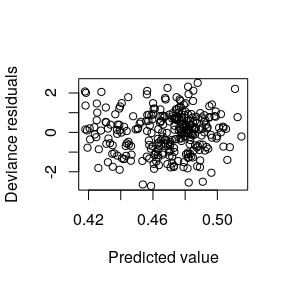# Introduction

The unifed distribution is the Exponential Dispersion Family (EDF) generated by the uniform distribution (see Jørgensen 1997 Chapters 2 and 3 to see how an EDF can be generated from a distribution). It has support on the interval (0,1).

The unifed R package focuses on the case where the dispersion parameter is equal to one. This is due to some numerical problems encountered when trying to implement the general case (see Quijano Xacur 2019 for details). The package also includes code for using this distribution with stan.

The unifed can be used as the response distribution of a GLM. Note that although the functions in this package implement the case with dispersion 1, it is still possible to have classes with weights different than one in a unifed GLM. This is because for a GLM we only need to minimize the deviance and disregard the rest of the density (which is the part that gives numerical stability).

We start with a section about Exponential Dispersion Families and their properties. Then we introduce the unifed density, it’s cumulant generator, and unit deviance function. This is followed by an example, where we use the package for fitting a frequentist and a Bayesian unifed GLM to a publicly available dataset. We close with a brief comparison between the unifed GLM and the beta regression.

# Exponential Dispersion Families

An Exponential Dispersion Family (EDF) is a set of distributions whose densities are given by

$\begin{equation} f(y|\theta,\phi) = a(y,\phi)\exp\left(\frac{1}{\phi} \left\{y\theta - \kappa(\theta) \right\}\right),\qquad \theta\in\Theta, \phi\in\Phi. \end{equation}$

$$\theta$$ and $$\Theta$$ are called the canonical parameter and canonical space, respectively and $$\phi$$ is known as the dispersion parameter. The parametrization above is know as the canonical parametrization. For$$\theta\in\mbox{int} \left( \Theta\right)$$ (here int stands for interior), $\mathbb{E}[Y] = \dot{\kappa}\left(\theta\right)\qquad \mbox{and}\qquad \mathbb{V}[Y]=\phi\ddot{\kappa}\left(\theta\right),$ where $$\dot{\kappa}=\kappa'$$ and $$\ddot{\kappa}=\dot{\kappa}'$$. This motivates the following definitions.

Definition Given an exponential dispersion family, the mean domain of the family is defined as $\Omega = \left\{\mu = \dot{\kappa}\left(\theta\right) : \theta \in \textrm{int}\left(\Theta\right) \right\}.$

Another important property is that the support of the distribution only depends on $$\phi$$ (and not on $$\theta$$). For a given family, let $$C_\phi$$ be the convex support of any member of the family with dispersion parameter $$\phi$$. We define the convex support of the family as $C_\Phi=\bigcup_{\phi\in\Phi} C_\phi.$

Definition The unit deviance function of an exponential dispersion family is defined as$$d: C_\Phi\times \Omega \rightarrow [0,\infty)$$ with $d\left(y,\mu\right) = 2 \left[ \sup_{\theta\in\Theta}\{\theta y - \kappa (\theta)\} - y \dot{\kappa}^{-1}(\mu) + \kappa\big(\dot{\kappa}^{-1}(\mu)\big) \right].$

The unit deviance function allows to re-parametrize the densities of the family as $\begin{equation} \label{eq:mean-value-par} f(y|\mu,\phi) = c(y,\phi)\exp\left(-\frac{1}{2\phi}d(y,\mu) \right) \end{equation}$ for some function $$c$$. This is known as the mean–value parametrization.

## Weights and Data Aggregation

In many applications it is useful to include a known positive weight to each observation. When this is done, the dispersion parameter is divided by the weight $$w$$, and the canonical and mean–value parametrizations become \begin{align*} f(y|\theta,\phi) &= a(y,\phi/w)\exp\left(\frac{w}{\phi} \left\{y\theta - \kappa(\theta) \right\}\right),\\ f(y|\mu,\phi) &= c(y,\phi/w)\exp\left(-\frac{w}{2\phi}d(y,\mu) \right). \end{align*}

There is a useful property of reproductive exponential dispersion families that allows for data aggregation. Jørgensen’s notation (from Jørgensen 1997) is very convenient to express this property: given a fixed exponential family, if $$Y$$ belongs to that family with mean $$\mu$$, dispersion parameter $$\phi$$ and weight $$w$$, we say that it is $$ED(\mu,\phi/w)$$ distributed. The property is then as follows: if $$Y_1,Y_2,\cdots,Y_n$$ are independent, and $$Y_i \sim ED(\mu,\phi/w_i)$$, then $\begin{equation} \label{eq:aggregate-equation} \bar{Y}=\frac{w_1Y_1+\cdots+w_nY_n}{w_+}\sim ED(\mu,\phi/w_+),\qquad w_+=\sum_{i=1}^n w_i. \end{equation}$

# The Unifed Family

The unifed can be characterized as the only EDF containing the uniform distribution. In fact, unifed = uniform + edf

Due to the numerical instability (see Quijano Xacur 2019) of the density when the dispersion parameter is different than 1, we focus here in the case where the dispersion parameter is equal to 1. In this case, the density is given by

$f(x;\theta) = \left\{ \begin{array}{ll} \frac{\theta}{e^\theta - 1} e^{x \theta} & \mbox{if } \theta \neq 0\\ 1 & \mbox{if } \theta = 0 \end{array} \right. \quad \mbox{for } x \in (0,1),$

where $$\theta$$ is called the canonical parameter. The unifed package provides the functions dunifed, punifed, qunfied and runifed for the density, distribution, quantile and random generation functions respectively. Here are some examples of use of these functions taken from the documentation

library(unifed)
dunifed( c(0.1,0.3,0.7), 10)

x <- c(0.1,0.4,0.7,1)
punifed(x,-5)

p <- 1:9/10
qunifed(p,5)

runifed(20,-3.3)

The mean of this distribution can be any value in (0,1). In the next a section explicit formula for the mean in terms of $$\theta$$ is given. A with any proper exponential family, there is a one-to-one correspondence between the mean $$\mu$$ and $$\theta$$. The next graphs show the density of the unifed as the mean varies.# Cumulant generator

The cumulant generator function characterizes and exponential family. For the unifed it is given by

$\kappa(\theta)=\left\{ \begin{array}{ll} \log\left(\frac{e^\theta-1}{\theta}\right)& \mbox{if }\theta\neq 0\\ 0 & \mbox{if }\theta=0 \end{array} \right..$

One difficulty in implementing $$\kappa$$ in a computer function is that $$e^\theta$$ above takes the value infinity even for relatively small $$\theta$$. For instance exp(1000) in R gives Inf.

To avoid this problem the function that implements $$\kappa$$ in the package, unifed.kappa, uses an approximation for values of $$\theta$$ that are greater than 50. This function is implemented as follows

$\kappa_{mod}(\theta)=\left\{ \begin{array}{ll} \kappa(\theta) & \mbox{if }\theta \leq 50\\ \theta - \log(\theta) & \mbox{if }\theta > 50 \end{array} \right..$

The justification for this approximation is that if $$y$$ is defined as $y = \log\left(\frac{e^\theta - 1 }{\theta}\right),$

then also,

$y = \theta - \log( \theta + e^{-y}).$

Now, $$\kappa$$ is an increasing function and $$y$$ goes to infinity as $$\theta$$ goes to infinity. Thus for large values of $$\theta$$ the term $$e^{-y}$$ is close to zero. We use 50 as the threshold for the approximation since evaluating the following code in R already gives zero

 log( ( exp(50) - 1) / 50  ) - ( 50 - log (50) )

# The Variance and Unit Deviance Functions

From the properties of exponential families we know then that if $$X$$ follows a unifed distribution distribution with canonical parameter $$\theta$$, its mean and variance are given by

\begin{aligned} \mathbb{E}[X]&= \dot{\kappa}(\theta) = \left\{ \begin{array}{ll} \frac{(\theta-1)e^\theta + 1}{\theta(e^\theta -1)} & \mbox{if }\theta \neq 0\\ \frac{1}{2} & \mbox{if }\theta=0 \end{array} \right., \qquad \\ \mathbb{V}[X]&= \ddot{\kappa}(\theta)=\left\{ \begin{array}{ll} \left(\frac{ e^{2\theta} - (\theta+2)e^\theta + 1} {\theta^2 (e^\theta-1)^2}\right)& \mbox{if }\theta \neq 0 \\ \frac{1}{12} & \mbox{if }\theta=0. \end{array} \right. \end{aligned}

Where $$\dot{\kappa}$$ and $$\ddot{\kappa}$$ are the first and second derivative of $$\kappa$$, respectively. The variance function allows us to express the variance in terms of the mean. It is defined as

${\bf V}(\mu) = \ddot{\kappa}(\dot{\kappa}^{-1}(\mu)).$

We have not been able to find an analytical expression for $$\dot{\kappa}^{-1}(\mu)$$. Thus, the function unifed.kappa.prime.inverseimplements it using the Newton-Raphson method for solving equations. It takes a value between 0 and 1 and it returns the value of $$\theta$$ that corresponds to that mean. There are some numerical stability problems around 0 and 0.5.

In order to solve the problem around 0.5, this function returns 0 (which is the image of 0.5), for every $$\mu$$ with $$|\mu - 0.5|<0.0001$$.

To address the problems around 0, unifed.kappa.prime.inverse returns $$-\dot{\kappa}^{-1}(1-\mu)$$ for $$\mu<0.1$$. The justification for this is that for every $$\theta$$

$\dot{\kappa}(\theta) = 1 - \dot{\kappa}(-\theta).$

In the package the variance function is implemented by the function unifed.varf. The following code plots of the values of the variance function.

x <- seq(0,1,length.out=100)
y <- unifed.varf(x)
plot(x,y,type="l",xlab=expression(mu),ylab=expression(bold(V)(mu)),main="Unifed Variance Function")We do not have an analytical expression for the unit deviance. The package provides the function unifed.deviance that computes the deviance numerically. It uses unifed.kappa.prime.inverse and the fact that the deviance can be expressed as

$d\left(y,\mu\right) = 2 \left[ y\{ \dot{\kappa}^{-1}(y) -\dot{\kappa}^{-1}(\mu) \} - \kappa\big(\dot{\kappa}^{-1}(y)\big) + \kappa\big(\dot{\kappa}^{-1}(\mu)\big) \right].$

# Unifed GLM - An Illustrative Example

The package provides the function unifed which returns a family that can be used with the glm function of R. This section gives an example of how to prepare a dataset and fit a unifed GLM.

The data from this section appears in Jong and Heller (2008). It is based on 67,856 one–year auto in- surance policies from 2004 or 2005. It can be downloaded from the companion site of the book, as the dataset called Car. The following text is the description of the variables provided by the website of the book

This data set is based on  one-year vehicle insurance
policies taken out in 2004 or 2005. There are 67856 policies, of
which  4624 (6.8%) had at least one claim.

Variables:

veh_value   vehicle value, in $10,000s exposure 0-1 clm occurrence of claim (0 = no, 1 = yes) numclaims number of claims claimcst0 claim amount (0 if no claim) veh_body vehicle body, coded as BUS CONVT = convertible COUPE HBACK = hatchback HDTOP = hardtop MCARA = motorized caravan MIBUS = minibus PANVN = panel van RDSTR = roadster SEDAN STNWG = station wagon TRUCK UTE - utility veh_age age of vehicle: 1 (youngest), 2, 3, 4 gender gender of driver: M, F area driver's area of residence: A, B, C, D, E, F agecat driver's age category: 1 (youngest), 2, 3, 4, 5, 6 We are interested in modeling the exposure; which is the proportion of time in a year in which the insurance policy is in-force for a given client. We use gender, agecat, area and veh_age as the explanatory variables. ## Downloading and Preparing the Data The following line of code downloads a csv file containing the data and stores in the file car.csv in your working folder. download.file("http://www.businessandeconomics.mq.edu.au/__data/assets/file/0011/232310/car.csv",destfile="car.csv") The following block reads and aggregates the data using data frames car.data <- read.csv("car.csv") car.data$agecat <- factor(car.data$agecat) car.data$veh_age <- factor(car.data$veh_age) agg.car.data <- aggregate( cbind(exposure,rep(1, dim(car.data) )) ~ gender + agecat + area + veh_age, data=car.data, FUN=sum) colnames(agg.car.data)[colnames(agg.car.data) == "V2"] <- "weight" colnames(agg.car.data)[colnames(agg.car.data) == "exposure"] <- "class.exposure" agg.car.data$class.exposure <- agg.car.data$class.exposure / agg.car.data$weight

Using data.table the same can be achieved with the following

library(data.table)
car.data[,agecat:=factor(agecat)]
car.data[,veh_age:=factor(veh_age)]

agg.car.data <- car.data[,.(class.exposure=sum(exposure)/.N,
weight=.N),
by=.(gender,agecat,area,veh_age)]

## Fitting and Diagnostics

Now that we have an aggregated version of our data in the variable agg.car.data, we can fit a unifed GLM as follows

exposure.model <- glm(class.exposure ~ gender + agecat + area + veh_age,
family=unifed(),
weights=weight,
data=agg.car.data)

The default link function for the unifed family is the logistic function. Since no argument as passed to unifed above it is the one being here. The documentation of unifed contains the other functions that can be used as link function and how to select them.

To see the glm summary of this model, we use the summary function. For the unifed family, it is necessary to pass the argument dispersion=1 explicitly. Otherwise we would be getting information for a unifed quasi family.

summary(exposure.model)
##
## Call:
## glm(formula = class.exposure ~ gender + agecat + area + veh_age,
##     family = unifed(), data = agg.car.data, weights = weight)
##
## Deviance Residuals:
##     Min       1Q   Median       3Q      Max
## -2.7196  -0.6981   0.1040   0.7400   2.5148
##
## Coefficients:
##              Estimate Std. Error z value Pr(>|z|)
## (Intercept) -0.331898   0.019711 -16.838  < 2e-16 ***
## genderM      0.028770   0.008995   3.198 0.001382 **
## agecat2      0.001109   0.018361   0.060 0.951818
## agecat3      0.053024   0.017834   2.973 0.002947 **
## agecat4      0.058287   0.017770   3.280 0.001038 **
## agecat5      0.104217   0.018921   5.508 3.63e-08 ***
## agecat6      0.069233   0.020958   3.303 0.000955 ***
## areaB        0.023933   0.013491   1.774 0.076057 .
## areaC        0.001392   0.012120   0.115 0.908564
## areaD        0.005330   0.015666   0.340 0.733668
## areaE        0.011977   0.017545   0.683 0.494834
## areaF        0.087916   0.021438   4.101 4.11e-05 ***
## veh_age2     0.170794   0.013775  12.398  < 2e-16 ***
## veh_age3     0.161287   0.013262  12.162  < 2e-16 ***
## veh_age4     0.154869   0.013429  11.533  < 2e-16 ***
## ---
## Signif. codes:  0 '***' 0.001 '**' 0.01 '*' 0.05 '.' 0.1 ' ' 1
##
## (Dispersion parameter for unifed family taken to be 1)
##
##     Null deviance: 585.47  on 287  degrees of freedom
## Residual deviance: 297.86  on 273  degrees of freedom
## AIC: NA
##
## Number of Fisher Scoring iterations: 3

The following shows a plot of the deviance residuals for this model

plot(predict(exposure.model, type="response"),
residuals(exposure.model, type="deviance"),
xlab="Predicted value",
ylab="Deviance residuals")## Verifying Data Aggregation

We mentioned before that GLMs allow for data aggregation. We use the example from the previous section to show explicitly what that means.

Let us fit the glm again but without aggregating the data:

exposure.model.2 <- glm(exposure ~ gender + agecat + area + veh_age,
family=unifed(),
data=car.data)

If you see the coefficients of the model above and compare them to the coefficients of the model in the previous section you will see that there are practically the same. Thus, when we said that the unifed GLM is suitable for data aggregation we meant that the fitted coefficients are the same for the original and the aggregated data. Note that this property is not exclusive for the unifed GLM. It is true for every GLM where the response is a continuous distribution.

Now, not all the printed decimals of the coefficients of both models are the same. This is because the algorithm for fitting glms stops after a convergence tolerance condition in the variation of the coefficients between iterations is met. If we want to decrease the variation between both models we can simply make the tolerance condition more strict by using the control argument of the glm function for both models:

exposure.model <- glm(class.exposure ~ gender + agecat + area + veh_age,
family=unifed(),
weights=weight,
control=list(epsilon=1e-20,maxit=1e5),
data=agg.car.data)

exposure.model.2 <- glm(exposure ~ gender + agecat + area + veh_age,
family=unifed(),
control=list(epsilon=1e-20,maxit=1e5),
data=car.data)

## Bayesian Unifed GLM

The package provides stan code for working with the unifed. It includes functions for computing the density and cumulative distribution function, random number generator, the cumulant generator and two of it’s derivatives. See the section unifed.stan in the manual of the package to see a comprehensive list. If you would like to get familiar with stan you could start with this tutorial.

We show now how to fit a Bayesian version of the unifed GLM example shown above in stan. For this we use the function unifed_glm_lp, which receives three arguments: a vector with the observed responses, the vector of canonical parameters and a vector of weights.

First save the following stan code in a file unifed_example.stan. Note that a normal distribution with mean 0 and standard deviation 20 is used as the prior of the regression coefficients.

functions{
#include unifed.stan
}

data{
int<lower=1> M; //Rows in the design matrix
int<lower=1> P; //Columns in the design matrix
matrix[M,P] X;
vector<lower=0,upper=1>[M] y;
int<lower=1> ws[M]; //Number of observations in each class
}

parameters{
vector[P] beta;
}

transformed parameters{
vector[M] theta;
theta = X*beta;
}

model{
beta ~ normal(0,20);
unifed_glm_lp(y, theta , to_vector(ws));
}

generated quantities{
vector[M] replicated_samples=rep_vector(0,M);
vector[M] mu;

for(i in 1:M){
int Nobs;
Nobs=ws[i];
for(n in 1:Nobs ){
replicated_samples[i]+=unifed_rng(theta[i]);
}
replicated_samples[i]/=Nobs;
mu[i]=unifed_kappa_prime(theta[i]);
}}

The include statement makes reference to the stan file provided in the unifed function. When the code is compiled we have to tell stan where to find that file. The R functions unifed.stan.path and unifed.stan.folder return the full path to the file and to the folder containing it respectively.

We now format data for the stan code. For this we use the variable agg.car.data defined before.

X <- model.matrix( ~ gender + agecat + area + veh_age, agg.car.data)

model.data <- list(M=dim(X),
P=dim(X),
X=X,
y= agg.car.data$class.exposure, ws= agg.car.data$weight)

Finally, the following code compiles the stan code, gets the simulations and saves them in the variable m1.

library(rstan)
model.list <- stanc_builder("unifed_example.stan",
isystem=unifed.stan.folder())

m1 <- stan(model_code=model.list\$model_code,
data=model.data,
warmup=3e3,
iter=2e4,
chains=4)

# Comparison to the Beta Regression

The beta regression (Ferrari and Cribari-Neto 2004) is a model for applications with a response variable in (0,1). In R it is implemented in the package betareg (Cribari-Neto and Zeileis 2010). That package includes the enhancements of the model from Simas, Barreto-Souza, and Rocha (2010), where explanatory variables are also used for the dispersion parameter.

The density of the beta distribution is much more flexible than the density of the unifed distribution. To see this compare the plot of the unifed densities shown here with the one provided for the beta in Ferrari and Cribari-Neto (2004).

In the cases where both a unifed GLM and a beta regression give a similar fit, the parsimony principle suggests to take the unifed GLM since it has less parameters.

On the computational side, when using an unifed GLM one can apply the properties of reproductive EDFs for data reduction.